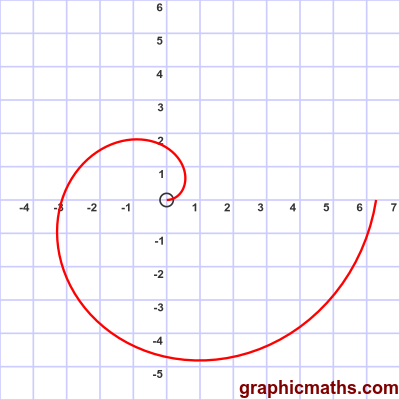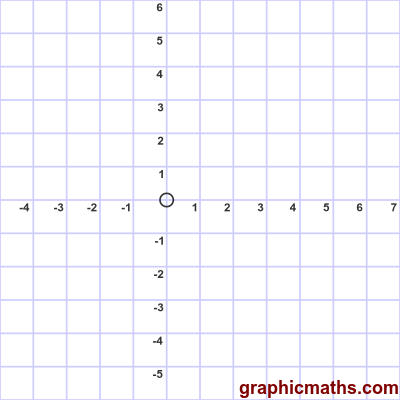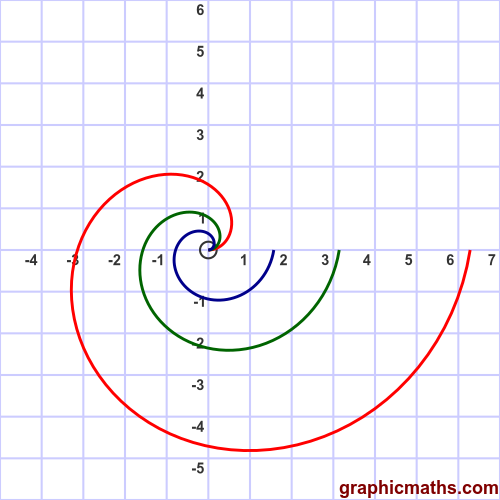# Polar curve r proportional to theta

Martin McBride, 2020-09-18
Tags standard curves
Categories polar coordinates

Here is a polar function where $r$ is proportional to $\theta$.

$$r = \theta$$

Describes a curve where $r$ is constant for any angle $\theta$, in this case 3. The curve includes all the points that are a distance of exactly 3 from the origin. This curve, of course, is a circle of radius 3:This animation shows how the curve is plotted as $\theta$ moves from 0 to $2\pi$:## Scaling the curve

In general the function:

$$r = a \theta$$

where $a$ is a non-negative constant, describes a spiral curve of that is similar to the curve above (ie it is the same shape, but scaled by $a$). Here are the curves created for $a = 1$ (red), $a = 1/2$ (green), and $a = 1/4$ (blue):The curve will cross the x-axis at 0, $-a\pi$ and $2 a\pi$. It will cross the y-axis at $a \frac{\pi}{2}$ and $-3 a \frac{\pi}{2}$.